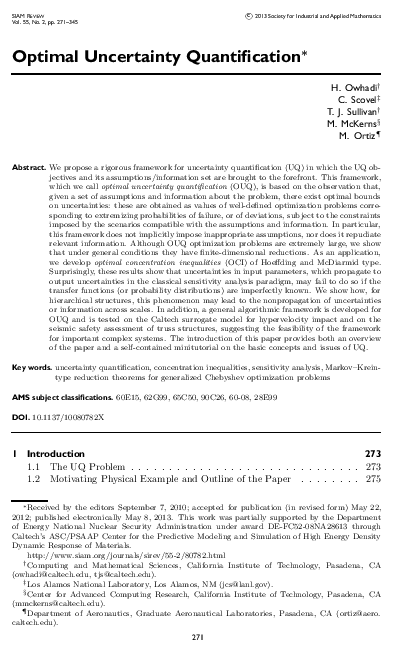# Tim Sullivan

### #ortiz

Clear Search### UQ for Legacy Data from Lipschitz Functions in M2AN

Mathematical Modelling and Numerical Analysis has just published a paper by Mike McKerns, Dominic Meyer, Florian Theil, Houman Owhadi, Michael Ortiz, and myself on optimal UQ for legacy data observations of Lipschitz functions.

In this paper, we address both mathematically and numerically the challenge of giving optimal bounds on quantities of interest of the form $$\mathbb{P}_{X \sim \mu}[f(X) \geq t]$$, where the probability distribution $$\mu$$ of $$X$$ is only partially known through some of its moments, and the forward model $$f$$ is partially known through some pointwise observations and smoothness information.

T. J. Sullivan, M. McKerns, D. Meyer, F. Theil, H. Owhadi, and M. Ortiz. “Optimal uncertainty quantification for legacy data observations of Lipschitz functions.” ESAIM. Mathematical Modelling and Numerical Analysis 47(6):1657–1689, 2013. doi:10.1051/m2an/2013083

Abstract. We consider the problem of providing optimal uncertainty quantification (UQ) – and hence rigorous certification – for partially-observed functions. We present a UQ framework within which the observations may be small or large in number, and need not carry information about the probability distribution of the system in operation. The UQ objectives are posed as optimization problems, the solutions of which are optimal bounds on the quantities of interest; we consider two typical settings, namely parameter sensitivities (McDiarmid diameters) and output deviation (or failure) probabilities. The solutions of these optimization problems depend non-trivially (even non-monotonically and discontinuously) upon the specified legacy data. Furthermore, the extreme values are often determined by only a few members of the data set; in our principal physically-motivated example, the bounds are determined by just 2 out of 32 data points, and the remainder carry no information and could be neglected without changing the final answer. We propose an analogue of the simplex algorithm from linear programming that uses these observations to offer efficient and rigorous UQ for high-dimensional systems with high-cardinality legacy data. These findings suggest natural methods for selecting optimal (maximally informative) next experiments.

Published on Friday 30 August 2013 at 18:00 UTC #publication #m2an #ouq #ortiz #owhadi #mckerns #meyer #theil### Optimal Uncertainty Quantification in SIAM Review

The 2013 Q2 issue of SIAM Review will carry an article by Houman Owhadi, Clint Scovel, Mike McKerns, Michael Ortiz, and myself on the optimization approach to uncertainty quantification in the presence of infinite-dimensional epistemic uncertainties about the probability measures and response functions of interest.

We present both a mathematical framework for the reduction of such infinite-dimensional problems to finite-dimensional effective feasible sets, and apply the methods to practical examples arising in hypervelocity impact and seismic safety certification.

H. Owhadi, C. Scovel, T. J. Sullivan, M. McKerns, and M. Ortiz. “Optimal Uncertainty Quantification.” SIAM Review 55(2):271–345, 2013. doi:10.1137/10080782X

Abstract. We propose a rigorous framework for uncertainty quantification (UQ) in which the UQ objectives and its assumptions/information set are brought to the forefront. This framework, which we call optimal uncertainty quantification (OUQ), is based on the observation that, given a set of assumptions and information about the problem, there exist optimal bounds on uncertainties: these are obtained as values of well-defined optimization problems corresponding to extremizing probabilities of failure, or of deviations, subject to the constraints imposed by the scenarios compatible with the assumptions and information. In particular, this framework does not implicitly impose inappropriate assumptions, nor does it repudiate relevant information. Although OUQ optimization problems are extremely large, we show that under general conditions they have finite-dimensional reductions. As an application, we develop optimal concentration inequalities (OCI) of Hoeffding and McDiarmid type. Surprisingly, these results show that uncertainties in input parameters, which propagate to output uncertainties in the classical sensitivity analysis paradigm, may fail to do so if the transfer functions (or probability distributions) are imperfectly known. We show how, for hierarchical structures, this phenomenon may lead to the nonpropagation of uncertainties or information across scales. In addition, a general algorithmic framework is developed for OUQ and is tested on the Caltech surrogate model for hypervelocity impact and on the seismic safety assessment of truss structures, suggesting the feasibility of the framework for important complex systems. The introduction of this paper provides both an overview of the paper and a self-contained minitutorial on the basic concepts and issues of UQ.

Published on Monday 10 June 2013 at 20:00 UTC #publication #siam-review #ouq #owhadi #scovel #mckerns #ortiz